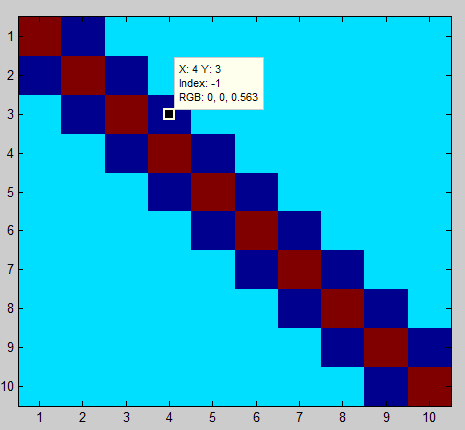# Flow control in Matlab using IF

## Introduction

This is the second lecture on what is called "control flow" in programming. We will use IF statements to control what Matlab excecutes.

## IF statemens

Help on IF statements can be found here.
```if/elseif/else

Execute statements if condition is true
Syntax
if expression
statements
elseif expression
statements
else
statements
end
Description
if expression, statements, end evaluates an expression, and
executes a group of statements when the expression is true.

elseif and else are optional, and execute statements only when
previous expressions in the if block are false.
An if block can include multiple elseif statements.
```

## Example 1

```>> a = 1;
>> if a==1
disp('Hello World')
end

Hello World
```

## Example 2

```>> b = 10;
>> if b == 10
disp('Value is exactly 10')
else
disp('Value is not 10, try again')
end

Value is exactly 10
```
```>> b = 5;
>> if b == 10
disp('Value is exactly 10')
else
disp('Value is not 10, try again')
end

Value is not 10, try again
```

## Example 3

Here is a sample script which demonstrates more complex flow control.
```X=ones(10);

if length(X)>500
disp('That is a long vector')
elseif length(X)==1
X=ones(500);
else
end
```
Video explanation:

## Example 4

This example for IF statements and For loops comes directly from the Matlab documentation on IF.
```% Preallocate a matrix
nrows = 10;
ncols = 10;
myData = ones(nrows, ncols);

% Loop through the matrix
for r = 1:nrows
for c = 1:ncols

if r == c
myData(r,c) = 2;
elseif abs(r - c) == 1
myData(r,c) = -1;
else
myData(r,c) = 0;
end

end
end

imagesc(myData)
```Video explanation:

## Example applied to Yellowstone Earthquakes

This example applies to a question from last class: How to control plotting of text.
```       if length(locations)~=0
s = sprintf('%2.1f',mean_depth);
text(long, lat, s);
end
```
Video explanation:

## Assignment 7: Japan Earthquake Analysis - Gridding and Image Plotting

For this assignment, assemble the operations of from the tutorials of "for loops" and image plotting using the Yellowstone seismicity data. Apply it to these data from 2011 from northern Japan:
japaneqs.txt. Note that the column order is different from that of the Yellowstone data. I downloaded them from this site: http://www.ncedc.org/cnss/catalog-search.html. Cut and paste the commands from the lecture into a Matlab m-file (script). Include comments in the script that tell the user what each group of commands is doing. Use functions to keep your code organized.

Build a web page for this assignment that contains the following:
1. A plot of magnitude versus time. What does it show? Note that the time is decimal days from the beginning of 2011.
2. (3D Plot) Plot 3D locations of earthquakes. Choose a good view orientation
3. For 3 different resolutions (stepsize = 0.5, 1, and 2 degrees)):
• (Grid Plot) Plot blue circles at each point in the grid on top of earthquake locations
• (Text Label Plot) Plot text labels for mean depths on top of earthquake locations. Use the IF statement so we don't see NaN plotted!
• (Scaled Image Plot) Plot scaled image (imagesc or pcolor) of mean depths below earthquake locations
4. A link to your M-files (Matlab script and functions) used to make these plots
5. Discussion: How does this seismicity pattern demonstrate the behavior of the subduction zone earthquakes: what is their time variationand what is the geometry of the seismicity cloud? How does that geometry relate to the tectonic setting?
• Web page for the assignment--5 points
• Time magnitude plot--5 points
• 3D Location Plot--5 points
• Grid Plots--5 points
• Text Label Plots--5 points
• Scaled Image Plots--5 points
• Matlab m-file (including comments)--10 points